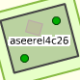# Am I wrong or there is a Bug on Turn Restriction on iD ?

 1 I think I found a bug on setting turn restrictions on iD in a particular point. The point is {Node id=1425835107 version=1 V lat=-8.0672848,lon=-34.8937994} The origin way of the restriction is {Way id=315073917 version=1 VT nodes=[{Node id=1425835107 version=1 V lat=-8.0672848,lon=-34.8937994}, {Node id=1425835109 version=1 V lat=-8.0671797,lon=-34.8940289}]} The destination way is {Way id=315073914 version=1 VT> nodes=[{Node id=1425835107 version=1 V lat=-8.0672848,lon=-34.8937994}, {Node id=1425835097 version=1 V lat=-8.0667292,lon=-34.8935357}, {Node id=1425830470 version=1 V lat=-8.0662714,lon=-34.8933326}]} The wrong way is {Way id=315073913 version=1 VT> nodes=[{Node id=1425835109 version=1 V lat=-8.0671797,lon=-34.8940289}, {Node id=1425835103 version=1 V lat=-8.0675578,lon=-34.8942197}, {Node id=1425835101 version=1 V lat=-8.06768,lon=-34.8942707}, {Node id=1425835082 version=1 V lat=-8.0677632,lon=-34.8942329}, {Node id=1425835088 version=1 V lat=-8.0678048,lon=-34.8941151}, {Node id=1425835108 version=1 V lat=-8.0677792,lon=-34.8940317}, {Node id=1425835107 version=1 V lat=-8.0672848,lon=-34.8937994}]} Every time I try to select the orign way and set the prohibition to turn to destination way, the iD selects the wrong way, so I can't set the restriction. asked 29 Nov '14, 23:38TrvrHldr 26●1●1●2 accept rate: 0%aseerel4c26 ♦ 32.6k●18●248●554 I had to ask a similar related question. But for clarification and also to see if it's the same issue: Now looking at the current map without looking at the streetview or any current turn restrictions, if you're traveling eastbound on Rua Francisco Alves, you can turn right on Miguel de Cervantes, but not right on Praça Miguel Cervantes (left is okay). Likewise westbound on Rua Francisco Alves, you can turn right on Praça Miguel Cervantes but not on Miguel de Cervantes (left is okay). Are these observations correct -- and if they are, it would imply that turn restrictions aren't needed because I can deduce them by looking at the map. I think that iD also prohibits adding these restrictions even if there is a sign on the street. Hopefully there's some clarification if a restriction or other annotation is needed in OSM for this situation. (10 Jul '19, 17:35) gpserror
Be the first one to answer this question!
 toggle preview community wiki:

By Email:

Markdown Basics

• *italic* or _italic_
• **bold** or __bold__
• image?![alt text](/path/img.jpg "title")
• numbered list: 1. Foo 2. Bar
• to add a line break simply add two spaces to where you would like the new line to be.
• basic HTML tags are also supported

Question tags:

×300
×45

question asked: 29 Nov '14, 23:38

question was seen: 2,095 times

last updated: 10 Jul '19, 17:35